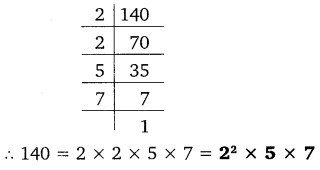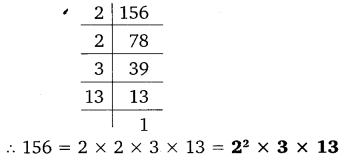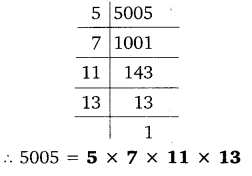# CLASS 10 MATH NCERT SOLUTIONS FOR CHAPTER – 1 REAL NUMBERS EX – 1.2

## Real Numbers

Question 1.
Express each number as a product of its prime factors:

(i) 140
(ii) 156
(iii) 3825
(iv) 5005
(v) 7429

Solution:
(i)
By prime factorization, we get:(ii) By prime factorization, we get:(iii) By prime factorization, we get:(iv) By prime factorization, we get:(v) By prime factorization, we get:Question 2.
Find the LCM and HCF of the following pairs of integers and verify that LCM x HCF = Product of the two numbers:

(i) 26 and 91
(ii) 510 and 92
(iii) 336 and 54

Solution:
(i)
By prime factorisation, we get:
26 = 2 x 13
91 = 7 x 13
∴ HCF of 26 and 91 = 13
and LCM of 26 and 91 = 2 x 7 x 13
= 182
Now, HCF x LCM = 182 x 13 = 2366 … (i)
Product of numbers = 26 x 91 = 2366  … (ii)
From (i) and (ii), we get:
HCF x LCM = Product of number
Hence, verified.

(ii) By prime factorisation, we get:
510 = 2 x 3 x 5 x 17
92 = 2 x 2 x 23
∴ HCF of 510 and 92 = 2
and LCM of 510 and 92
= 22 x 3 x 5 x 17 x 23 = 23460
Now, HCF x LCM = 2 x 23460 = 46920 … (i)
Product of numbers
= 510 x 92 = 46920 … (ii)
From (i) and (ii), we get:
LCM x HCF = Product of numbers
Hence, verified.

(iii) By prime factorisation, we get:
336 = 2 x 2 x 2 x 2 x 3 x 7
54 = 2 x 3 x 3 x 3
∴ HCF of 336 and 54 = 2 x 3 = 6
and LCM of 336 and 54 = 24 x 33 x 7
= 3024
Now, LCM x HCF = 3024 x 6 = 18144… (i)
Product of numbers
= 336 x 54 = 18144 … (ii)
From (i) and (ii), we get:
LCM x HCF = Product of number
Hence, verified.

Question 3.
Find the LCM and HCF of the following integers by applying the prime factorisation method:

(i) 12, 15 and 21
(ii) 17, 23 and 29
(iii) 8, 9 and 25

Solution:
(i)
By prime factorisation, we get:
12 = 2 x 2 x 3
15 = 3 x 5
21 = 3 x 7
∴ HCF of 12, 15 and 21 = 3
and LCM = 2 x 2 x 3 x 5 x 7 = 420.

(ii)
By prime factorisation, we get:
17 = 17 x 1
23 = 23 x 1
29 = 29 x 1
∴ HCF of 17, 23 and 29 = 1
and LCM = 17 x 23 x 29 = 11339.

(iii) By prime factorisation, we get:
8=2 x 2 x 2 x 1
9 = 3 x 3 x 1
25 = 5 x 5 x 1
∴  HCF of 8, 9 and 25 = 1
and LCM of 8, 9 and 25 = 23 x 32 x 52
= 1800.

Question 4.
Given that HCF (306, 657) = 9, find LCM (306, 657).

Solution:
HCF (306, 657) = 9Hence, LCM (306, 657) = 22338.

Question 5.
Check whether 6n can end with the digit 0 for any natural number n.

Solution:
6n will end with 0 if 5 is one of the primes of 6.
Prime factors of 6 = 2 and 3.
Since 5 is not a prime factor of 6,
therefore, 6n cannot end with the digit 0.

Question 6.
Explain why 7 x 11 x 13 + 13 and 7 x 6 x 5 x 4 x 3 x 2 x 1 + 5 are composite numbers.

Solution:
7 x 11 x 13 + 13 = 13 x (7 x 11 + 1) = 13 x 78
The given number has more than two factors.
Hence, it is a composite number.
7 x 6 x 5 x 4 x 3 x 2 x 1+5
= 5 x (7 x 6 x 4 x 3 x 2 x 1 + 1)
= 5 x 1009 x 1
The given number has more than two factors.
Hence, it is a composite number.

Question 7.
There is a circular path around a sports field. Sonia takes 18 minutes to drive one round of the field, while Ravi takes 12 minutes for the same. Suppose they both start at the same point and at the same time and go in the same direction. After how many minutes will they meet again at the starting point?

Solution:
The minimum time when Sonia and Ravi meet again at starting point will be the LCM of 18 min and 12 min. LCM of 12 and 18 is 36.
Hence, they will meet each other at starting point after 36 min.

### Take Your IIT JEE Coaching to Next Level with Examtube

• Mentoring & Teaching by IITians
• Regular Testing & Analysis
• Preparation for Various Engineering Entrance Exams
• Support for School/Board Exams
• 24/7 Doubts Clarification